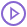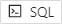# Fitting Road Network Data to a Graph

How to model a graph based on a road network dataset

The following guide provides step-by-step instructions to get started with fitting existing road network data to a graph. Since edges in graphs can only be composed of two nodes, the weight (or cost to travel) for complex WKT LINESTRINGs (e.g., more than two points) that typically define road networks must be assigned consistently to the segments composing the LINESTRING, as noted under Weights. There are two methods for calculating and assigning weights to a graph with edges derived from large WKT LINESTRINGs:

1. In-line Expressions -- calculating weights using inline expressions and forcing the graph server to divide complex WKT LINESTRINGs during the graph creation
2. Expansion -- using iteration to divide complex WKT LINESTRINGs and, using a subquery, calculate weights prior to the graph creation

## Prerequisites

### Data File

The tutorial makes use of the dc_shape dataset, which can be ingested from the data file mentioned above.

To ingest the file using Workbench:

1. Log in to Workbench; see Connecting to the Kinetica Cloud Workbench.
2. Click Import on the top menu.
3. Click File Upload to upload the file through KiFS and then import the data from it.
4. In the Setup step, enter the name of a KiFS directory as the Folder
5. For File, select the data file on your local file system to upload, then click Upload.
6. In the Source step, click Next.
7. In the Destination step, enter tutorial_graph for the Schema.
8. Ensure dc_shape is specified as the Table.
9. Click Import.

The file will be validated and records will be inserted.

## Fit Using Inline Expressions

Fitting road network data to a graph using inline expressions offers a simpler but less efficient approach to deriving the individual edge segments' weight. The dc_shape_inline graph that will be created will calculate weight as distance inline and is based on the aforementioned dc_shape dataset.

### Create Schema

Before starting with graph creation, a schema, tutorial_graph_fit_data, will be created to contain the tables supporting graph creation & solving. To create the schema:

1. On the left side under the Data tab, click the + next to Tables & Views and then click Add New Schema.
2. Enter input tutorial_graph_fit_data as the Name, and click Create.

### Create Graph

To create the graph, in Workbench:

1. On the left side of the screen, click on Workbooks.
2. Click the + and select Add New Workbook.
3. Name your new workbook Fitting Data and click Create.
4. Copy the following CREATE GRAPH statement into the first block of your workbook:
 `````` 1 2 3 4 5 6 7 8 9 10 11 12 13 14 15 16 17 18 19 `````` ``````CREATE DIRECTED GRAPH tutorial_graph_fit_data.dc_shape_inline ( EDGES => INPUT_TABLE ( SELECT link_id AS ID, shape AS WKTLINE, direction AS DIRECTION, ST_Length(shape,1)/(ST_NPoints(shape)-1) AS WEIGHT_VALUESPECIFIED FROM tutorial_graph.dc_shape ), OPTIONS => KV_PAIRS ( 'merge_tolerance' = '0.00001', 'enable_graph_draw' = 'true', 'recreate' = 'true', 'graph_table' = 'tutorial_graph_fit_data.dc_shape_inline_graph' ) ) ``````
5. Click the run iconto create the inline graph.

This creates the dc_shape_inline graph with the following characteristics:

• It is DIRECTED because the roads in the graph have directionality (one-way and two-way roads).
• It has no explicitly defined nodes, because the example relies on implicit nodes attached to the defined edges.
• The EDGES are derived from WKT LINESTRINGs in the shape column of the dc_shape table (AS WKTLINE); the graph server will automatically divide each complex LINESTRING into simple edges. Each edge's directionality is derived from the direction column of the dc_shape table (AS DIRECTION), and each edge will be assigned an ID that is the link_id column of the dc_shape table (AS ID).
• The edge weights are represented as distance, which is calculated as the length of the entire shape column's LINESTRING (in meters) divided by the number of points in the LINESTRING minus 1 (AS WEIGHT_VALUESPECIFIED).
• It has no inherent restrictions for any of the nodes or edges in the graph.
• It utilizes the following OPTIONS:
• It will be replaced with this instance of the graph if a graph of the same name exists (recreate).
• If nodes are within 0.00001 degrees (1 meter) of each other, they are merged together (merge_tolerance).
• The resulting graph's information is placed into a table (enable_graph_draw/graph_table) and an EDGE_WKTLINE column is included so the graph can be visualized.

To view the created graph:

1. Click on dc_shape_inline under Graphs in the left hand menu, and click Preview.
2. Click Update to use the default map settings.

## Fit Using Expansion

Fitting road network data to a graph using expansion separates the creation of line segments and calculation of distance from graph creation. This increases the performance of the graph creation process, as most of the calculations are done within the database instead of the graph server. Expansion is achieved through iteration, wherein each source record with a WKT is expanded into a number of records equal to the number of edges in that corresponding WKT. For example, a single road record with a 10-segment WKT LINESTRING will become ten individual records, each containing one of the 10 segments. In this way, the weights can be pre-calculated and assigned directly to the road segments/edges, alleviating the need for the graph server to do this processing itself.

### Create Expansion Table

To accomplish this, first create the expansion table, in Workbench:

1. If not already viewing the Fitting Data workbook, click Explore at the top of the screen, and then click the Fitting Data workbook.
2. Click theicon to add a new SQL Block.
3. Copy the following into the new block:
 `````` 1 2 3 4 5 6 7 8 9 10 11 12 13 14 15 `````` ``````CREATE TABLE tutorial_graph_fit_data.dc_shape_expanded AS SELECT link_id, direction, REMOVE_NULLABLE(shape) AS shape, REMOVE_NULLABLE(ST_Length(shape,1)) AS distance FROM ( SELECT direction, link_id, ST_MakeLine(ST_PointN(shape, i + 1), ST_PointN(shape, i + 2)) AS shape FROM tutorial_graph.dc_shape JOIN ITER ON i < ST_NumPoints(shape) - 1 ) ``````
4. Click the run iconto create the expanded data table.

This CREATE TABLE...AS statement performs several actions necessary to expand the edges and prepare the data for use by the graph creation process.

First the complex LINESTRINGs are divided into single edges via a subquery:

• The existing dc_shape table is joined to the ITER virtual table, where each WKT LINESTRING is iterated through to extract line segments.
• A new WKT LINESTRING is constructed (ST_MakeLine) from each consecutive pair of points in the source LINESTRING (dc_shape.shape) column.
• The direction and link_id columns are extracted from the dc_shape table to assist in graph creation later.

Next, an outer query is used to eliminate the column nullability introduced by join (since graphs cannot be created from columns with the nullable property), and also to calculate the weight as distance. Lastly, the result is saved to a table, which the graph creation will use as input.

• The results of the query are placed in a new table (tutorial_graph_fit_data.dc_shape_expanded).
• The link_id and direction columns are passed through as they are.
• The shape column has its nullability removed (REMOVE_NULLABLE).
• The shape column is used to calculate a distance column (distance), and nullability is removed from that column as well.

### Create Graph from Expansion Table

Finally, the dc_shape_expansion graph is created directly from the dc_shape_expanded table with no extra calculations necessary. The graph is created with the same characteristics as before, with the following changes:

• The edges are derived from the line segments expanded from the original WKT LINESTRINGs in the shape column of the dc_shape_expanded table (WKTLINE).
• The weights are pulled directly from the distance column of the dc_shape_expanded table (WEIGHT_VALUESPECIFIED), pre-calculated above.

To create the graph:

1. Click theicon to add another new SQL Block.
2. Copy the following into the new block:
 `````` 1 2 3 4 5 6 7 8 9 10 11 12 13 14 15 16 17 18 19 `````` ``````CREATE DIRECTED GRAPH tutorial_graph_fit_data.dc_shape_expansion ( EDGES => INPUT_TABLE ( SELECT shape AS WKTLINE, link_id AS ID, direction AS DIRECTION, distance AS WEIGHT_VALUESPECIFIED FROM tutorial_graph_fit_data.dc_shape_expanded ), OPTIONS => KV_PAIRS ( 'merge_tolerance' = '0.00001', 'enable_graph_draw' = 'true', 'recreate' = 'true', 'graph_table' = 'tutorial_graph_fit_data.dc_shape_expanded_graph' ) ) ``````
3. Click the run iconto create the graph from the expanded data table.

To view the created graph:

1. Click on dc_shape_expansion under Graphs in the left hand menu, and click Preview.
2. Click Update to use the default map settings.